Main Content

# Direction of Arrival Estimation with Beamscan, MVDR, and MUSIC

This example illustrates using beamscan, MVDR, and MUSIC for direction of arrival (DOA) estimation. Beamscan is a technique that forms a conventional beam and scans it over directions of interest to obtain a spatial spectrum. Minimum variance distortionless response (MVDR) is similar to beamscan but uses an MVDR beam. Multiple signal classification (MUSIC) is a subspace method that provides high resolution DOA estimates. For all three methods, the peaks of the output spatial spectrum indicate the DOAs of the received signals. In this example, we illustrate the use of beamscan, MVDR, and MUSIC to estimate broadside angles with a uniform linear array (ULA) and azimuth and elevation angles with a uniform rectangular array (URA).

### Modeling ULA's Received Signal

First, model a uniform linear array (ULA) containing 10 isotropic antennas spaced 0.5 meters apart.

```ula = phased.ULA('NumElements',10,'ElementSpacing',0.5); ```

Assume that two narrowband signals impinge on the array. The first signal arrives from 40° in azimuth and 0° in elevation, while the second signal arrives from -20° in azimuth and 0° in elevation. The operating frequency of the system is 300 MHz.

```ang1 = [40; 0]; % First signal ang2 = [-20; 0]; % Second signal angs = [ang1 ang2]; c = physconst('LightSpeed'); fc = 300e6; % Operating frequency lambda = c/fc; pos = getElementPosition(ula)/lambda; Nsamp = 1000; ```

Also, assume that the thermal noise power at each antenna is 0.01 Watts.

```nPower = 0.01; ```

Generate the multichannel signal received by the ULA.

```rs = rng(2007); signal = sensorsig(pos,Nsamp,angs,nPower); ```

### Beamscan DOA Estimation with a ULA

We want to estimate the two DOAs using the received signal. Because the signal is received by a ULA, which is symmetric around its axis, we cannot obtain both azimuth and elevation at the same time. Instead, we can estimate the broadside angle, which is measured from the broadside of the ULA. The relationship between these angles is illustrated in the following figure: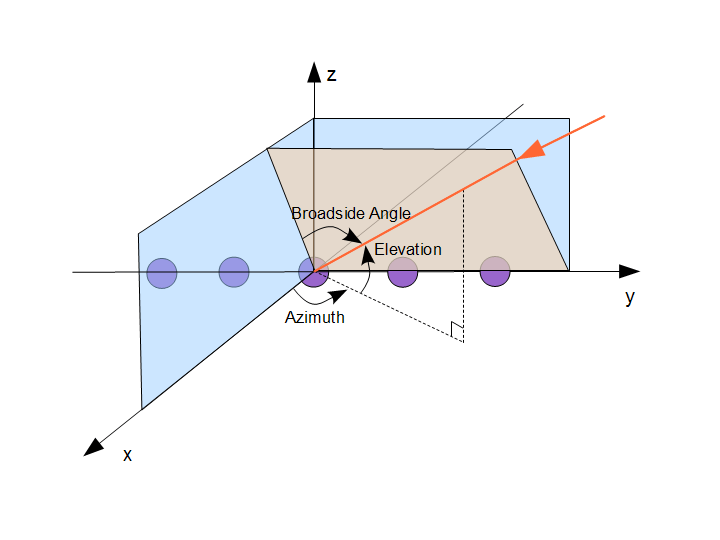The broadside angles corresponding to the two incident directions are:

```broadsideAngle = az2broadside(angs(1,:),angs(2,:)) ```
```broadsideAngle = 40.0000 -20.0000 ```

We can see that the two broadside angles are the same as the azimuth angles. In general, when the elevation angle is zero and the azimuth angle is within [-90 90], the broadside angle is the same as the azimuth angle. In the following we only perform the conversion when they are not equal.

The beamscan algorithm scans a conventional beam through a predefined scan region. Here we set the scanning region to [-90 90] to cover all 180 degrees.

```spatialspectrum = phased.BeamscanEstimator('SensorArray',ula,... 'OperatingFrequency',fc,'ScanAngles',-90:90); ```

By default, the beamscan estimator only produces a spatial spectrum across the scan region. Set the DOAOutputPort property to true to obtain DOA estimates. Set the NumSignals property to 2 to find the locations of the top two peaks.

```spatialspectrum.DOAOutputPort = true; spatialspectrum.NumSignals = 2; ```

We now obtain the spatial spectrum and the DOAs. The estimated DOAs show the correct values, which are 40° and -20°.

```[~,ang] = spatialspectrum(signal) ```
```ang = 40 -20 ```

Plot the spatial spectrum of the beamscan output.

```plotSpectrum(spatialspectrum); ```### Improving Resolution Using MVDR and MUSIC Estimators

The conventional beam cannot resolve two closely-spaced signals. When two signals arrive from directions separated by less than the beamwidth, beamscan will fail to estimate the directions of the signals. To illustrate this limitation, we simulate two received signals from 30° and 40° in azimuth.

```ang1 = [30; 0]; ang2 = [40; 0]; signal = sensorsig(pos,Nsamp,[ang1 ang2],nPower); [~,ang] = spatialspectrum(signal) ```
```ang = 35 71 ```

The results differ from the true azimuth angles. Let's take a look at the output spectrum.

```plotSpectrum(spatialspectrum); ```The output spatial spectrum has only one dominant peak. Therefore, it cannot resolve these two closely-spaced signals. When we try to estimate the DOA from the peaks of the beamscan output, we get incorrect estimates. The beamscan object returns two maximum peaks as the estimated DOAs no matter how different the peaks are. In this case, the beamscan returns the small peak at 71° as the second estimate.

To resolve closely-spaced signals, we can use the minimum variance distortionless response (MVDR) algorithm or the multiple signal classification (MUSIC) algorithm. First, we will examine the MVDR estimator, which scans an MVDR beam over the specified region. Because an MVDR beam has a smaller beamwidth, it has higher resolution.

```mvdrspatialspect = phased.MVDREstimator('SensorArray',ula,... 'OperatingFrequency',fc,'ScanAngles',-90:90,... 'DOAOutputPort',true,'NumSignals',2); [~,ang] = mvdrspatialspect(signal) plotSpectrum(mvdrspatialspect); ```
```ang = 30 40 ```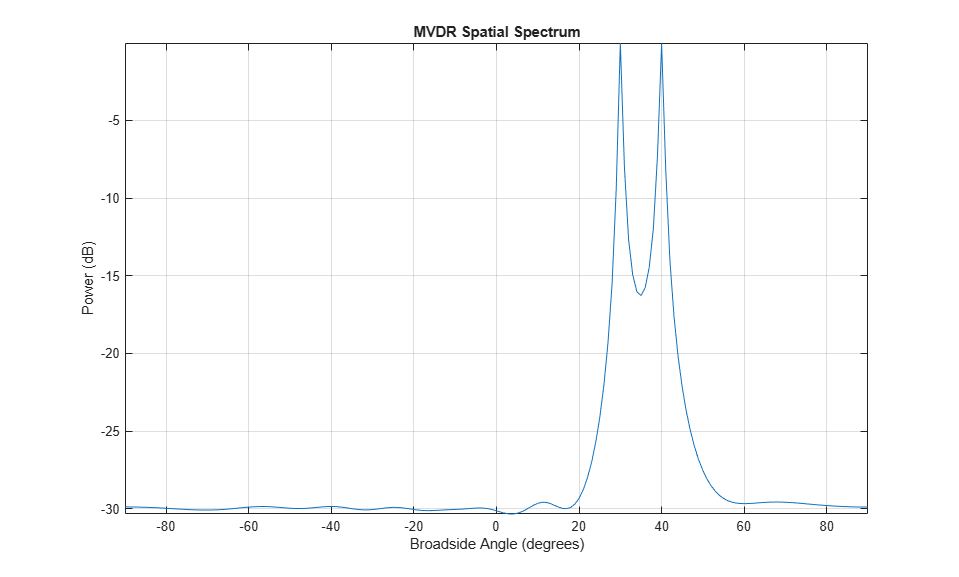The MVDR algorithm correctly estimates the DOAs that are unresolvable by beamscan. The improved resolution comes with a price. The MVDR is more sensitive to sensor position errors. In circumstances where sensor positions are inaccurate, MVDR could produce a worse spatial spectrum than beamscan. Moreover, if we further reduce the difference of two signal directions to a level that is smaller than the beamwidth of an MVDR beam, the MVDR estimator will also fail.

The MUSIC algorithm can also be used to resolve these closely-spaced signals. Estimate the directions of arrival of the two sources and compare the spatial spectrum of MVDR to the spatial spectrum of MUSIC.

```musicspatialspect = phased.MUSICEstimator('SensorArray',ula,... 'OperatingFrequency',fc,'ScanAngles',-90:90,... 'DOAOutputPort',true,'NumSignalsSource','Property','NumSignals',2); [~,ang] = musicspatialspect(signal) ymvdr = mvdrspatialspect(signal); ymusic = musicspatialspect(signal); helperPlotDOASpectra(mvdrspatialspect.ScanAngles,... musicspatialspect.ScanAngles,ymvdr,ymusic,'ULA') ```
```ang = 30 40 ```The directions of arrival using MUSIC are correct, and MUSIC provides even better spatial resolution than MVDR. MUSIC, like MVDR, is sensitive to sensor position errors. In addition, the number of sources must be known or accurately estimated. When the number of sources specified is incorrect, MVDR and Beamscan may simply return insignificant peaks from the correct spatial spectrum. In contrast, the MUSIC spatial spectrum itself may be inaccurate when the number of sources is not specified correctly. In addition, the amplitudes of MUSIC spectral peaks cannot be interpreted as the power of the sources.

For a ULA, additional high resolution algorithms can further exploit the special geometry of the ULA. See High Resolution Direction of Arrival Estimation.

### Converting Broadside Angles to Azimuth

Although we can only estimate broadside angles using a ULA, we can convert the estimated broadside angles to azimuth angles if we know their incoming elevations. We now model two signals coming from 35° in elevation and estimate their corresponding broadside angles.

```ang1 = [40; 35]; ang2 = [15; 35]; signal = sensorsig(pos,Nsamp,[ang1 ang2],nPower); [~,ang] = mvdrspatialspect(signal) ```
```ang = 32 12 ```

The resulting broadside angles are different from either the azimuth or elevation angles. We can convert the broadside angles to the azimuth angles if we know the elevation.

```ang = broadside2az(ang,35) ```
```ang = 40.3092 14.7033 ```

### Beamscan DOA Estimation with a URA

Next, we illustrate DOA estimation using a 10-by-5 uniform rectangular array (URA). A URA can estimate both azimuth and elevation angles. The element spacing is 0.3 meters between each row, and 0.5 meters between each column.

```ura = phased.URA('Size',[10 5],'ElementSpacing',[0.3 0.5]); ```

Assume that two signals impinge on the URA. The first signal arrives from 40° in azimuth and 45° in elevation, while the second signal arrives from -20° in azimuth and 20° in elevation.

```ang1 = [40; 45]; % First signal ang2 = [-20; 20]; % Second signal signal = sensorsig(getElementPosition(ura)/lambda,Nsamp, ... [ang1 ang2],nPower); rng(rs); % Restore random number generator ```

Create a 2-D beamscan estimator object from the URA. This object uses the same algorithm as the 1-D case except that it scans over both azimuth and elevation instead of broadside angles.

The scanning region is specified by the property 'AzimuthScanAngles' and 'ElevationScanAngles'. To reduce computational complexity, we assume some a priori knowledge about the incoming signal directions. We restrict the azimuth scan region to [-45 45] and the elevation scan region to [10 60].

```azelspectrum = phased.BeamscanEstimator2D('SensorArray',ura,... 'OperatingFrequency',fc,... 'AzimuthScanAngles',-45:45,'ElevationScanAngles',10:60,... 'DOAOutputPort',true,'NumSignals',2); ```

The DOA output is a 2-by-N matrix where N is the number of signal directions. The first row contains azimuth angles while the second row contains elevation angles.

```[~,ang] = azelspectrum(signal) ```
```ang = 40 -20 45 20 ```

Plot a 3-D spectrum in azimuth and elevation.

```plotSpectrum(azelspectrum); ```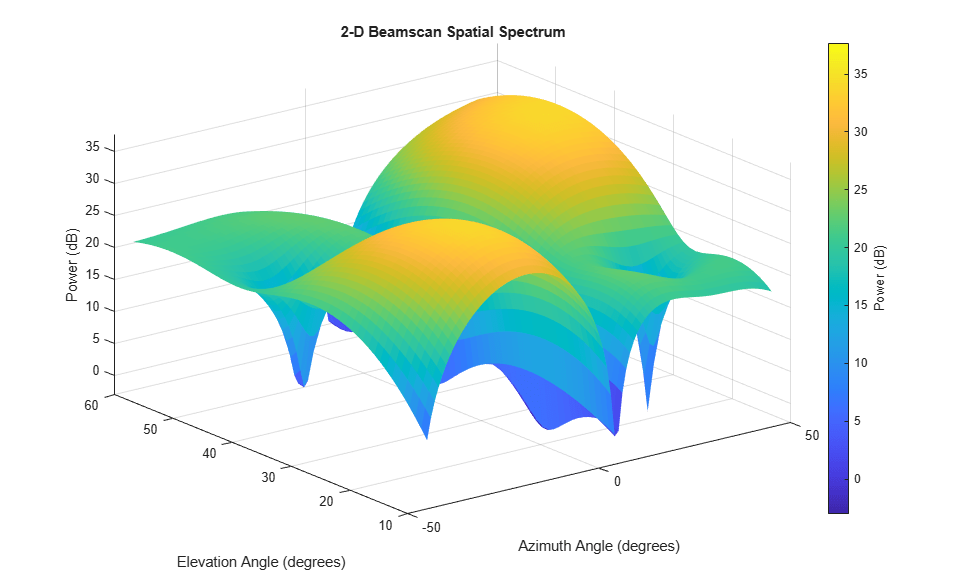### MVDR DOA Estimation with a URA

Similar to the ULA case, we use a 2-D version of the MVDR algorithm. Since our knowledge of the sensor positions is perfect, we expect the MVDR spectrum to have a better resolution than beamscan.

```mvdrazelspectrum = phased.MVDREstimator2D('SensorArray',ura,... 'OperatingFrequency',fc,... 'AzimuthScanAngles',-45:45,'ElevationScanAngles',10:60,... 'DOAOutputPort',true,'NumSignals',2); [~,ang] = mvdrazelspectrum(signal) plotSpectrum(mvdrazelspectrum); ```
```ang = -20 40 20 45 ```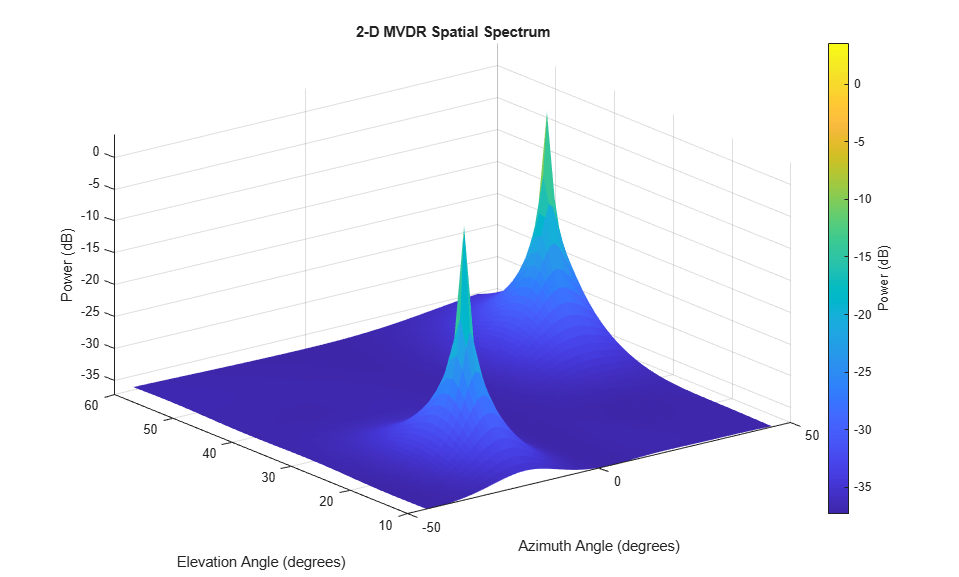### MUSIC DOA Estimation with a URA

We can also use the MUSIC algorithm to estimate the directions of arrival of the two sources.

```musicazelspectrum = phased.MUSICEstimator2D('SensorArray',ura,... 'OperatingFrequency',fc,... 'AzimuthScanAngles',-45:45,'ElevationScanAngles',10:60,... 'DOAOutputPort',true,'NumSignalsSource','Property','NumSignals',2); [~,ang] = musicazelspectrum(signal) plotSpectrum(musicazelspectrum); ```
```ang = -20 40 20 45 ```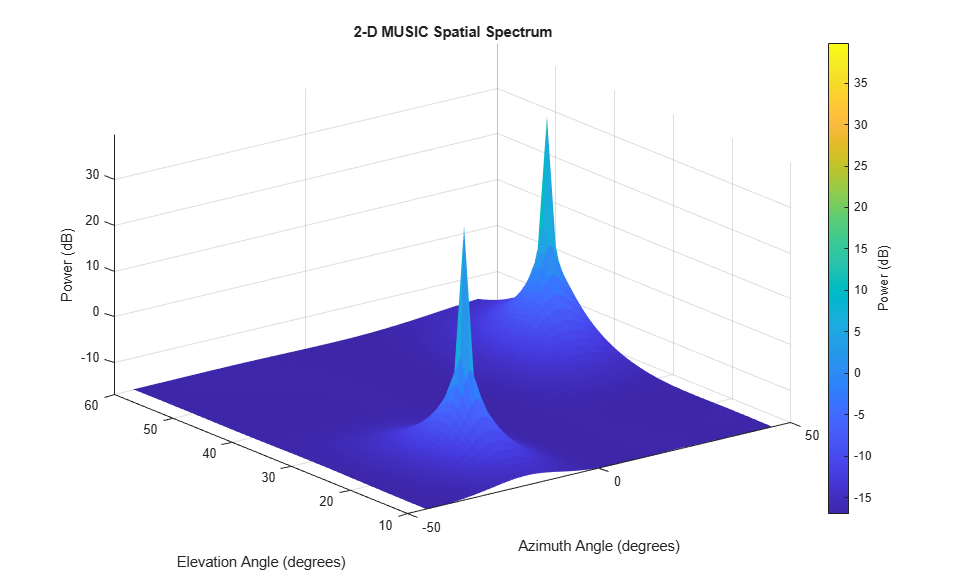To compare MVDR and MUSIC estimators, let's consider sources located even closer together. Using MVDR and MUSIC, compute the spatial spectrum of two sources located at 10° in azimuth and separated by 3° in elevation.

```ang1 = [10; 20]; % First signal ang2 = [10; 23]; % Second signal signal = sensorsig(getElementPosition(ura)/lambda,Nsamp, ... [ang1 ang2],nPower); [~,angmvdr] = mvdrazelspectrum(signal) [~,angmusic] = musicazelspectrum(signal) ```
```angmvdr = 10 -27 22 21 angmusic = 10 10 23 20 ```

In this case, only MUSIC correctly estimates to directions of arrival for the two sources. To see why, plot an elevation cut of each spatial spectrum at 10° azimuth.

```ymvdr = mvdrazelspectrum(signal); ymusic = musicazelspectrum(signal); helperPlotDOASpectra(mvdrazelspectrum.ElevationScanAngles,... musicazelspectrum.ElevationScanAngles,ymvdr(:,56),ymusic(:,56),'URA') ```Since the MUSIC spectrum has better spatial resolution than MVDR, MUSIC correctly identifies the sources while MVDR fails to do so.

### Summary

In this example, we showed how to apply the beamscan, MVDR, and MUSIC techniques to the DOA estimation problem. We used both techniques to estimate broadside angles for the signals received by a ULA. The MVDR algorithm has better resolution than beamscan when there is no sensor position error. MUSIC has even better resolution than MVDR, but the number of sources must be known. We also illustrated how to convert between azimuth and broadside angles. Next, we applied beamscan, MVDR, and MUSIC to estimate both azimuth and elevation angles using a URA. In all of these cases, we plotted the output spatial spectrum, and found again that MUSIC had the best spatial resolution. Beamscan, MVDR, and MUSIC are techniques that can be applied to any type of array, but for ULAs and URAs there are additional high resolution techniques that can further exploit the array geometry.

## Support

#### Exploring Hybrid Beamforming Architectures for 5G Systems

Download white paper